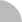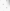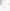(34.239.176.198) 您好！臺灣時間：2021/04/23 20:38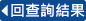### 詳目顯示:::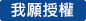: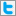Twitter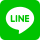•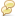被引用:0
•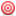點閱:161
•評分: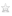•下載:0
•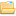書目收藏:0
 圖形G上的一條漢彌爾頓路徑是通過G上每一頂點恰好一次的簡單路徑。圖形G上的一條漢彌爾頓迴路則是通過G上每一頂點恰好一次的簡單迴路。漢彌爾頓路徑與漢彌爾頓迴路問題分別在判斷一個圖形是否存在一條漢彌爾頓路徑或漢彌爾頓迴路。漢彌爾頓問題包括漢彌爾頓路徑與漢彌爾頓迴路問題。一個圖形G上的路徑覆概是由一些頂點互斥的路徑所構成的集合，且此路徑覆概通過G上的所有頂點。路徑覆概問題在於找到一個最少路徑數的路徑覆概。因為判斷是否存在一個恰包含一條路徑的路徑覆概等效於漢彌爾頓路徑問題，因此，漢彌爾頓路徑問題是路徑覆概問題的一個特例。我們稱漢彌爾頓問題為路徑覆概相關問題。 在一般圖形上，路徑覆概及其相關問題是典型的NP-complete問題。因此，探討路徑覆概及其相關問題的研究者總是集中焦點於某些具有特殊性質的圖形上。完美圖是一個吸引釵h研究者專注的特殊圖形。在某些完美圖的特殊子集合圖形上，路徑覆概及其相關問題已被證明是NP-complete。然而，當輸入圖形是某些其它完美圖的特殊子集合圖形時，則這些問題可在多項式時間內找到最佳解。另一方面，某些研究者也有考慮非完美圖之特殊圖形上的路徑覆概及其相關問題。在此論文中，我們將集中焦點於解決保距圖與圓弧圖上的路徑覆概及其相關問題。保距圖形成完美圖的一個特殊子集合圖形，而圓弧圖則是非包含於完美圖的特殊圖形。 若一個圖形的任兩個頂點與其在任何包含此兩頂點的延生子圖等距，則稱此圖形為保距圖。一個圖形G的頂點集合若可以表示成圓上的圓弧集合，且兩個圓弧若相交則其所代表的頂點在G中即有邊相連，則稱此圖形為圓弧圖。圓弧圖上的漢彌爾頓迴路問題已被證明可在多項式時間內解決。保距圖上的漢彌爾頓問題已被證明可在多項式時間內解決，但這些圖形上的路徑覆概問題之複雜度仍然未知。 在此論文中，我們首先提出一個O(n)時間的近似演算法，此處輸入的圓弧圖為n個端點已排序過的圓弧集合。我們也證明此近似演算法所找到的頂點互斥路徑數比最佳解最多多一條路徑。使用此結果，我們可在O(n)時間內，將圓弧圖上的路徑覆概問題轉化為漢彌爾頓問題。因此，圓弧圖上路徑覆概問題的複雜度與漢彌爾頓問題相同。接著，我們提出一個一致性的方法，在線性時間內解決保距圖上的漢彌爾頓問題。此部份改進了目前已知的最好結果。最後，我們將提出第一個多項式時間演算法來解決保距圖上的路徑覆概問題。
 A Hamiltonian path of a graph G is a simple path that visits each vertex of G exactly once. A Hamiltonian cycle of a graph is a simple cycle with the same property. The Hamiltonian path and Hamiltonian cycle problems involve testing whether a Hamiltonian path and a Hamiltonian cycle exist in a graph, respectively. The Hamiltonian problems include the Hamiltonian path and Hamiltonian cycle problems. A path cover of a graph G is a set of pairwise vertex-disjoint paths of G that covers all vertices in G. The path cover problem is to find a path cover of the minimum number of paths of a graph. The path cover problem contains the Hamiltonian path problem as a special case since finding a path cover, consisting of a single path, corresponds directly to the Hamiltonian path problem. The Hamiltonian problems are called the path cover related problems. It is well known that the path cover and related problems for general graphs are classic NP-complete problems. Hence researchers studying the path cover and related problems always focus on special classes of graphs. Perfect graphs have received much attentions. The path cover and related problems on some special classes of perfect graphs have been shown to be NP-complete. However, they admit polynomial-time algorithms when the input is restricted to be in some other special classes of perfect graphs. On the other hand, some researchers also considered some special classes of graphs not in perfect graphs for the path cover and related problems. In the dissertation, we will focus on solving the path cover and related problems on distance-hereditary graphs and circular-arc graphs. Distance-hereditary graphs form a subclass of perfect graphs, but circular-arc graphs are not contained in the class of perfect graphs. A graph is a distance-hereditary graph if each pair of vertices is equidistant in every connected induced subgraph containing them. A graph G is a circular-arc graph if there exist a set F of arcs on a circle and a one-to-one mapping of the vertices of G and the arcs in F such that two vertices in G are adjacent if and only if their corresponding arcs in F intersect. The Hamiltonian cycle problem on circular-arc graphs has been shown to be polynomially solvable. The Hamiltonian problems on distance-hereditary graphs have been shown to be solvable in polynomial time, but the complexity of the path cover problem on these graphs is still unknown. In this dissertation, we first present a simple O(n)-time approximation algorithm for the path cover problem on circular-arc graphs given a set of n arcs with endpoints sorted and show that the cardinality of the path cover found by the approximation algorithm is at most one more than the optimal one. Using the result, we reduce the path cover problem on circular-arc graphs to the Hamiltonian problems on the same class of graphs in O(n) time. Hence the complexity of the path cover problem on circular-arc graphs is the same as those of the Hamiltonian problems on circular-arc graphs. Next we present a unified approach to solving the Hamiltonian problems on distance-hereditary graphs in linear time. This improves the best known results. Finally, we propose the first polynomial-time algorithm to solve the path cover problem on distance-hereditary graphs.
 AbstractTable of ContentsList of FiguresList of Tables1. Introduction2. Preliminaries 2.1. Graph Classes 2.1.1. Containment Relations among Graph Classes 2.1.2. Circular-Arc Graphs 2.1.3. Distance-Hereditary Graphs 2.1.4. Bounded Clique-Width Graphs 2.2. A Brief Survey on the Path Cover and Related Problems 2.3. Reducing the Path Cover Problem to the Hamiltonian Problems 2.4. Terminology3. The Path Cover Problem on Circular-Arc Graphs 3.1. Notation and Definitions 3.2. A Greedy Algorithm for the Path Cover Problem on Interval Graphs 3.3. An Approximation Algorithm on Circular-Arc Graphs 3.4. Reducing the Path Cover Problem to the Hamiltonian Problems4. The Hamiltonian Problems on Distance-Hereditary Graphs 4.1. Notation and Definitions 4.2. The Hamiltonian Path Problem on Distance-Hereditary Graphs 4.3. The Other Hamiltonian Problems5. The Path Cover Problem on Distance-Hereditary Graphs 5.1. Notation and Definitions 5.2. Properties on the Path Cover Problem 5.3. A Dynamic Programming Polynomial-Time Algorithm6. Conclusions and Future ResearchBibliographyVitaPublication ListsResearch Projects
  T. Akiyama, T. Nishizeki, and N. Saito, NP-completeness of the Hamiltonian cycle problem for bipartite graphs, J. Inform. Process. 3 (1980/81) 73--76. S.G. Akl, Parallel Computation: Models and Methods (Prentice-Hall, Englewood Cliffs, NJ, 1997). S.G. Akl and B.K. Bhattacharya, Computing maximum cliques of circular arcs in parallel, Parallel Algorithms Appl. 12(1997) 305--320. M.O. Albertson, Finding Hamiltonian cycles in Ore graphs, Congr. Numer. 58 (1987) 25--27. M.G. Andrews and D.T. Lee, Parallel algorithms on circular-arc graphs, Comput. Geom. 5 (1995) 117--141. S.R. Arikati and C. Pandu Rangan, Linear algorithm for optimal path cover problem on interval graphs, Inform. Process.Lett. 35 (1990) 149--153. S.R. Arikati, C. Pandu Rangan, and G.K. Manacher, Efficient reduction for path problems on circular-arc graphs, BIT 31 (1991) 182--193. N. Ascheuer, Hamiltonian path problems in the on-line optimization of flexible manufacturing systems, Technique Report TR96-3, Konrad-Zuse-Zentrum fur Informationstechnik, Berlin,1996. M.J. Atallah, D.Z. Chen, and D.T. Lee, An optimal algorithm for shortest paths on weighted interval and circular-arc graphs, with applications, Algorithmica 14 (1995) 429--441. H.J. Bandelt and H.M. Mulder, Distance-hereditary graphs, J. Combin. Theory Ser. B 41 (1986) 182--208. H.J. Bandelt, A. Henkmann, and F. Nicolai, Powers of distance-hereditary graphs, Discrete Math. 145 (1995)37--60. J.C. Bermond, Hamiltonian graphs, in Selected Topics in Graph Theory ed. by L.W. Beinke and R.J. Wilson (Academic Press,New York, 1978). C. Berge, Farbung von graphen, deren samtliche bzw. deren ungerade Kreise starr sind, Wiss. Z. Martin-Luther-Univ., Halle-Wittenberg Math.-Natur, Reihe (1961)  A.A. Bertossi and M.A. Bonuccelli, Hamiltonian circuits in interval graph generalizations, Inform. Process. Lett. 23(1986) 195--200. S. Bespamyatnikh, B. Bhattacharya, M. Keil, D. Kirkpatrick, and M. Segal, Efficient algorithms for centers and medians in interval and circular-arc graphs, Networks 39 (2002) 144--152. F.T. Boesch and J.F. Gimpel, Covering the points a digraph with point-disjoint paths and its application to code optimization, J. ACM 24 (1977) 192--198. M.A. Bonuccelli and D.P. Bovet, Minimum node disjoint path covering for circular-arc graphs, Inform. Process. Lett. 8 (1979) 159--161. K.S. Booth and G.S. Lueker, Testing for the consecutive ones property, interval graphs and graph planarity using PQ-tree algorithms, J. Comput. System Sci. 13 (1976) 355--379. A. Brandstädt and F.F. Dragan, A linear-time algorithm for connected r-domination and Steiner tree on distance-hereditary graphs, Networks 31 (1998) 177--182. A. Brandstädt, V.B. Le, and J.P. Spinrad, Graph Classes: A Survey (SIAM Monographs on Discrete Mathematics and Applications, Philadelphia, 1999). A. Brandstädt, F.F. Dragan, and E. Köhler, Linear time algorithms for Hamiltonian problems on (Claw, Net)-free graphs, SIAM J. Comput. 30 (2000) 1662--1677. A. Brandstädt and V.V. Lozin, On the linear structure and clique-width of bipartite permutation graphs, Ars Combin. 67 (2003) 273--281. H.J. Broersma, E. Dahlhaus, and T. Kloks, A linear time algorithm for minimum fill-in and treewidth for distance hereditary graphs, Discrete Appl. Math. 99 (2000) 367--400. M.S. Chang, S.L. Peng, and J.L. Liaw, Deferred-query – an efficient approach for some problems on interval and circular-arc graphs, in: Proceedings of the 3rd Workshop on Algorithms and Data Structures (WADS’93), Lecture Notes in Comput. Sci., vol. 709, Springer, Berlin/New York, 1993, pp. 222--233. M.S. Chang, S.Y. Hsieh, and G.H. Chen, Dynamic programming on distance-hereditary graphs, in: Proceedings of the 8thAnnual International Symposium on Algorithms and Computation (ISAAC’97), Lecture Notes in Comput. Sci., vol. 1350,Springer, Berlin/New York, 1997, pp. 344--353. M.S. Chang, Efficient algorithms for the domination problems on interval and circular-arc graphs, SIAM J. Comput. 27(1998) 1671--1694. M.S. Chang and R.L. Yang, Edge domination of distance-hereditary graphs, in: Proceedings of the Workshop on Algorithms, International Computer Symposium (ICS’98), Taiwan, 1998, pp. 112--115. M.S. Chang, S.L. Peng, and J.L. Liaw, Deferred-query: an efficient approach for some problems on interval graphs, Networks 34 (1999) 1--10. M.S. Chang, S.C. Wu, G.J. Chang, and H.G. Yeh, Hamiltonian problems on Ptolemaic graphs, in: Proceedings of the Workshop on Algorithms and Theory of Computation, International Computer Symposium (ICS’2000), Taiwan, 2000, pp.27--34. M.S. Chang, S.C. Wu, G.J. Chang, and H.G. Yeh, Domination in distance-hereditary graphs, Discrete Appl. Math. 116(2002) 103--113. G.J. Chang and D. Kuo, The L(2,1)-labeling problem on graphs, SIAM J. Comput. 9 (1996) 309--316. G.J. Chang, Corrigendum to: The path-partition problem in block graphs, Inform. Process. Lett. 83 (2002) 293. R.S. Chang, Jump number maximization for proper interval graphs and series-parallel graphs, Inform. Sci. 115 (1999) 103--122. L. Chen, Efficient parallel recognition of some circular arc graphs. II, Algorithmica 17 (1997) 266--280. L. Chen, Optimal circular arc representations: properties, recognition, and construction, J. Comput. System Sci. 56 (1998) 320--331 D.Z. Chen, D.T. Lee, R. Sridhar, and C.N. Sekharan, Solving the all-pair shortest path query problem on interval and circular-arc graphs, Networks 31 (1998) 249--258. N. Chiba and T. Nishizeki, The Hamiltonian cycle problem is linear-time solvable for 4-connected plannar graphs, J. Algorithms 10 (1989) 187--211. V. Chvátal and P.L. Hammer, Set-patching and threshold, Res. Report CORR-73-21, University of Waterloo, 1973. S. Cicerone and G. Di Stefano, Graph classes between parity and distance-hereditary graphs, Discrete Appl. Math. 95(1999) 197--216. S. Cicerone, G. Di Stefano, and M. Flammini, Compact-port routing models and applications to distance-hereditary graphs, J. Parallel Distrib. Comput. 61 (2001) 1472--1488. S. Cicerone and G. Di Stefano, On the extension of bipartite to parity graphs, Discrete Appl. Math. 95 (1999) 181--195. D.G. Corneil, H. Lerchs, and L.K. Stewart, Complement reducible graphs, Discrete Appl. Math. 3 (1981) 163--174. D.G. Corneil, Y. Perl, and L.K. Stewart, A linear recognition algorithm for cographs, SIAM J. Comput. 14 (1985) 926--934. D.G. Corneil, H. Lerchs, and L. Stewart Burlingham, Complement reducible graphs, Discrete Appl. Math. 3 (1994) 163--174. D.G. Corneil, M. Habib, J.M. Lanlignel, B. Reed, and U. Rotics, Polynomial time recognition of clique-width at most three graphs, in: Proceedings of the 4th Latin American Symposium on Theoretical Informatics (LATIN’2000)}, Lecture Notes in Comput. Sci., vol. 1776, Springer, Berlin/New York, 2000, pp.126--134. B. Courcelle, J. Engelfriet, and G. Rozenberg, Handle-rewriting hypergraph grammars, J. Comput. System Sci. 46 (1993) 218--270. B. Courcelle and S. Olariu, Upper bounds to the clique width of graphs, Discrete Appl. Math. 101 (2000) 77--114. B. Courcelle, J.A. Makowsky, and U. Rotics, Linear time solvable optimization problems on graphs of bounded clique-width, Theory Comput. Syst. 33 (2000) 125--150. E. Dahlhaus, P. Dankelmann, W. Goddard, and H.C. Swart, MAD trees and distance-hereditary graphs, Discrete Appl. Math. 131(2003) 151--167. P. Damaschke, The Hamiltonian circuit problem for circle graphs is NP-complete, Inform. Process. Lett. 32 (1989) 1--2. P. Damaschke, J.S. Deogun, D. Kratsch, and G. Steiner, Finding Hamiltonian paths in cocomparability graphs using the bump number algorithm, Order 8 (1992) 383--391. P. Damaschke, Paths in interval graphs and circular arc graphs, Discrete Math. 112 (1993) 49--64. G. Damiand, M. Habib, and C. Paul, A simple paradigm for graph recognition: application to cographs and distance-hereditary graphs, Theoret. Comput. Sci. 263 (2001) 99--111. J.S. Deogun and G. Steiner, Polynomial algorithms for Hamiltonian cycle in cocomparability graphs, SIAM J. Comput. 23(1994) 520--552. J.S. Deogun and C. Riedesel, Hamiltonian cycles in permutation graphs, J. Combin. Math. Combin. Comput. 27 (1998) 161--200. J.S. Deogun, T. Kloks, D. Kratsch, and H. Müller, On the vertex ranking problem for trapezoid, circular-arc and other graphs, Discrete Appl. Math. 98 (1999) 39--63 G. Di Stefano, A routing algorithm for networks based on distance-hereditary topologies, in: Proceedings of the 3rd International Colloquium on Structural Information and Communication Complexity (SIROCCO’96), Siena, Italy,1996, pp. 141--151. P.F. Dietz, Intersection graph algorithms, Ph.D. thesis, Comp. Sci. Dept., Cornell University, Ithaka, New York, 1984. F.F. Dragan, Dominating cliques in distance-hereditary graphs, in: Proceedings of the 4th Scandinavian Workshop on Algorithm Theory (SWAT’94), Lecture Notes in Comput. Sci., vol.824, Springer, Berlin/New York, 1994, pp. 370--381. F.F. Dragan and F. Nicolai, LexBFS-orderings of distance-hereditary graphs with application to the diametral pair problem, Discrete Appl. Math. 98 (2000) 191--207. E.M. Eschen and J.P. Spinrad, An O(n^2) algorithm for circular-arc graph recognition, in: Proceedings of the 4th Annual ACM-SIAM Symposium on Discrete Algorithm (SODA’93), 1993, pp. 128--137. A.H. Esfahanian and O.R. Oellermann, Distance-hereditary graphs and multidestination message-routing in multicomputers, J. Combin. Math. Combin. Comput. 13 (1993) 213--222. A.H. Esfahanian and O.R. Oellermann, Corrigendum to: Distance-hereditary graphs and multidestination message-routing in multicomputers, J. Combin. Math. Combin. Comput. 14 (1993) 221. W. Espelage, F. Gurski, and E. Wanke, How to solve NP-hard graph problems on clique-width bounded graphs in polynomial time, in: Proceedings of the 27th International Workshop on Graph-Theoretic Concepts in Computer Science (WG’2001), Lecture Notes in Comput. Sci., vol. 2204, Springer, Berlin/NewYork, 2001, pp. 117--128. T. Feder, P. Hell, and J. Huang, List homomorphisms and circular arc graphs, Combinatorica 19 (1999) 487--505 M.R. Garey, D.S. Johnson, and R.E. Tarjan, The planar Hamiltonian circuit problem is NP-complete, SIAM J. Comput. 5 (1976) 704--714. M.R. Garey and D.S. Johnson, Computers and Intractability: A Guide to the Theory of NP-Completeness (Freeman, San Francisco, CA, 1979). F. Gavril, Algorithms on circular-arc graphs, Networks 4 (1974) 357--369. M.U. Gerber and D. Kobler, Algorithms for vertex-partitioning problems on graphs with fixed clique-width, Theoret. Comput. Sci. 299 (2003) 719--734. M.C. Golumbic, Algorithmic Graph Theory and Perfect Graphs (Academic Press, New York, 1980). M.C. Golumbic and P.L. Hammer, Stability in circular arc graphs, J. Algorithms 9 (1988) 314--320. M.C. Golumbic and R.C. Laskar, Irredundancy in circular arc graphs, Discrete Appl. Math. 44 (1993) 79--89. M.C. Golumbic and U. Rotics, On the clique-width of some perfect graph classes, Internat. J. Found. Comput. Sci. 11 (2000) 423--443. S. Goodman and S. Hedetniemi, On the Hamiltonian completion problem, in: Proc. 1973 Capital Conf. on Graph Theory and Combinatorics, Lecture Notes in Math., vol. 406, Springer, Berlin, 1974, pp. 262--272. J.L. Gross and J. Yellen, Handbook of Graph Theory (CRC Press, New York, 2004). P.L. Hammer and B. Simeone, The splittance of a graph, Combinatorica 1 (1981) 275--284. P.L. Hammer and F. Maffray, Completely separable graphs, Discrete Appl. Math. 27 (1990) 85--99. P. Hell and J. Huang, Interval bigraphs and circular arc graphs, J. Graph Theory 46 (2004) 313--327. E. Howorka, A characterization of distance-hereditary graphs, Quart. J. Math. 28 (1977) 417--420. E. Howorka, A characterization of Ptolemaic graphs, J. Graph Theory 5 (1981) 323--331. S.Y. Hsieh, C.W. Ho, T.S. Hsu, M.T. Ko, and G.H. Chen, A faster implementation of a parallel tree contraction scheme and its application on distance-hereditary graphs, J. Algorithms 35 (2000) 50--81. S.Y. Hsieh, C.W. Ho, T.S. Hsu, and M.T. Ko, Efficient algorithms for the Hamiltonian problem on distance-hereditary graphs, in: Proceedings of the 8th Annual International Conference on Computing and Combinatorics (COCOON’2002), Lecture Notes in Comput. Sci., vol. 2387, Springer, Berlin/New York, 2002, pp. 77--86. S.Y. Hsieh, An efficient parallel strategy for the two-fixed-endpoint Hamiltonian path problem on distance-hereditary graphs, J. Parallel Distrib. Comput. 64 (2004) 662--685. W.L. Hsu and K.H. Tsai, Linear time algorithms on circular-arc graphs, Inform. Process. Lett. 40 (1991) 123--129. W.L. Hsu, O(M N) algorithms for the recognition and isomorphism problems on circular-arc graphs, SIAM J. Comput. 24 (1995) 411--439. W.L. Hsu and J.P. Spinrad, Independent sets in circular-arc graphs, J. Algorithms 19 (1995) 145--160. R.W. Hung and M.S. Chang, A simple linear algorithm for the connected domination problem in circular-arc graphs, Discuss. Math. Graph Theory 24 (2004) 137--145. R.W. Hung, S.C. Wu, and M.S. Chang, Hamiltonian cycle problem on distance-hereditary graphs, J. Inform. Sci. Eng. 19 (2003) 827--838. A. Itai, C.H. Papadimitriou, and J.L. Szwarcfiter, Hamiltonian paths in grid graphs, SIAM J. Comput. 11 (1982) 676--686. D.S. Johnson, The NP-complete Column: an Ongoing Guide, J. Algorithms 6 (1985) 434--451. H.A. Jung, On a class of posets and the corresponding comparability graphs, J. Combin. Theory Ser. B 24 (1978) 125--133. I.A. Karapetjan, Coloring of arc graphs (in Russian), Akad. Nauk Armjan. SSR Dokl. 70 (1980) 306--311. J.M. Keil, Finding Hamiltonian circuits in interval graphs, Inform. Process. Lett. 20 (1985) 201--206. J.M. Keil and D. Schaefer, An optimal algorithm for finding dominating cycles in circular-arc graphs, Discrete Appl. Math. 36 (1992) 25--34. T. Kloks, D. Kratsch, and C.K. Wong, Minimum fill-in on circle and circular-arc graphs, J. Algorithms 28 (1998) 272--289. D. Kobler and U. Rotics, Edge dominating set and colorings on graphs with fixed clique-width, Discrete Appl. Math. 126 (2003) 197--221. M.S. Krishnamoorthy, An NP-hard problem in bipartite graphs, SIGACT News 7 (1976) 26. V. Kumar, An approximation algorithm for circular arc colouring, Algorithmica 30 (2001) 406--417. T.H. Lai and S.S. Wei, Algorithms for page retrieval and Hamiltonian paths on forward-convex line graphs, J. Algorithms 18 (1995) 358--375. J. van Leeuwen, Graph Algorithms: Handbook of Theoretical Computer Science Vol. A (Elsevier, Amsterdam, 1990). Y.D. Liang and C. Rhee, Finding a maximum matching in a circular-arc graph, Inform. Process. Lett. 45 (1993) 185--190. Y.D. Liang and G.K. Manacher, An O(n logn) algorithm for finding a minimal path cover in circular-arc graphs, in: Proceedings of the ACM conference on Computer Science, Indianapolis, IN, USA, 1993, pp. 390--397. Y.D. Liang, G.K. Manacher, C. Rhee, and T.A. Mankus, A linear algorithm for finding Hamiltonian circuits in circular-arc graphs, in: Proceedings of the 32nd Southeast ACM conference, 1994, pp. 15--22. R. Lin, S. Olariu, and G. Pruesse, An optimal path cover algorithm for cographs, Comput. Math. Appl. 30 (1995) 75--83. T.H. Ma and J.P. Spinrad, On the 2-chain subgraph cover and related problems, J. Algorithms 16 (1994) 251--268. J.A. Makowsky and U. Rotics, On the clique-width of graphs with few P4’s, Internat. J. Found. Comput. Sci. 10 (1999) 329--348. G.K. Manacher, T.A. Mankus, and C.J. Smith, An optimumQ(n logn) algorithm for finding a canonical Hamiltonian path and a canonical Hamiltonian circuit in a set of intervals, Inform. Process. Lett. 35 (1990) 205--211. G.K. Manacher and T.A. Mankus, A simple linear time algorithm for finding a maximum independent set of circular arcs using intervals alone, Networks 39 (2002) 68--72. M.V. Marathe, H.B. Hunt, and S.S. Ravi, Efficient approximation algorithms for domatic partition and on-line coloring of circular arc graphs, Discrete Appl. Math. 64 (1996) 135--149. S. Masuda and K. Nakajima, An optimal algorithm for finding a maximum independent set of a circular-arc graph, SIAM J. Comput. 17 (1988) 41--52. R.M. McConnell and J.P. Spinrad, Linear-time transitive orientation, in: Proceedings of the 8th Annual ACM-SIAM Symposium on Discrete Algorithms (SODA’97), 1997, pp.19--25. R.M. McConnell, Linear-time recognition of circular-arc graphs, Algorithmica 37 (2003) 93--147. T.A. McKee, Restricted circular-arc graphs and clique cycles, Discrete Math. 263 (2003) 221--231. S. Moran and Y. Wolfstahl, Optimal covering of cacti by vertex disjoint paths, Theoret. Comput. Sci. 84 (1991) 179--197. S. Moran, I. Newman, and Y. Wolfstahl, Approximation algorithms for covering a graph by vertex-disjoint paths of maximum total weight, Networks 20 (1990) 55--64. H. Müller and F. Nicolai, Polynomial time algorithms for the Hamiltonian problems on bipartite distance-hereditary graphs, Inform. Process. Lett. 46 (1993) 225--230. H. Müller, Hamiltonian circuits in chordal bipartite graphs, Discrete Math. 156 (1996) 291--298. G. Narasimhan, A note on the Hamiltonian circuit problem on directed path graphs, Inform. Process. Lett. 32 (1989) 167--170. S. Natarajan and A.P. Sprague, Disjoint paths in circular arc graphs, Nordic J. Comput. 3 (1996) 256--270. F. Nicolai, Hamiltonian problems on distance-hereditary graphs, Technique Report SM-DU-264, Gerhard-Mercator University, Germany, 1994 (corrected version 1996). F. Nicolai and T. Szymczak, Homogeneous sets and dominations: a linear time algorithm for distance-hereditary graphs, Networks 37 (2001) 117--128. S.C. Ntafos and S. Louis Hakimi, On path cover problems in digraphs and applications to program testing, IEEE Trans. Software Engrg. 5 (1979) 520--529. J.F. O’Callaghan, Computing the perceptual boundaries of dot patterns, Comput. Graphics Image Process. 3 (1974) 141--162. R. Paige and R.E. Tarjan, Three partition refinement algorithms, SIAM J. Comput. 16 (1987) 973--989. S.S. Pinter and Y. Wolfstahl, On mapping processes to processors, Internat. J. Parallel Programming 16 (1987) 1--15. J. Plesník, The NP-completeness of the Hamiltonian cycle problem in bipartite cubic planar graphs, Acta Math. Univ. Comenian. 42/43 (1983) 271--273. F.P. Preperata and M.I. Shamos, Computational Geometry: An Introduction (Springer, New York, 1985). C. Riedesel and J.S. Deogun, Permutation graphs: Hamiltonian paths, J. Combin. Math. Combin. Comput. 19 (1995) 55--63. D.J. Rose, R.E. Tarjan, and G.S. Lueker, Algorithmic aspects of vertex elimination on graph, SIAM J. Comput. 5 (1976) 266--283. A. Saha, M. Pal, and T.K. Pal, An optimal parallel algorithm for solving all-pairs shortest paths problem on circular-arc graphs, J. Appl. Math. Comput. 17 (2005) 1--23. A.A. Schäffer, A faster algorithm to recognize undirected path graphs, Discrete Appl. Math. 43 (1993) 261--295. W.K. Shih, T.C. Chern, and W.L. Hsu, An O(n^2 logn) time algorithm for the Hamiltonian cycle problem on circular-arc graphs, SIAM J. Comput. 21 (1992) 1026--1046. Z. Skupień, Path partitions of vertices and Hamiltonicity of graphs, Recent Advances in Graph Theory, in: Proceedings of the 2nd Czechoslovak Symposium, Prague, 1974 (Academia, Prague, 1975) pp. 481--491. J.P. Spinrad, Two-dimentional partial orders, Ph.D.thesis, Dept. of EECS, Princeton University, NJ, 1982. J.P. Spinrad, On comparability and permutation graphs, SIAM J. Comput. 14 (1985) 658--670. J.P. Spinrad, A. Brandstädt, and L. Stewart, Bipartite permutation graphs, Discrete Appl. Math. 18 (1987) 279--291. J.P. Spinrad, Doubly lexical ordering of dense 0-1 matrices, Inform. Process. Lett. 45 (1993) 229--235. J.P. Spinrad, Recognition of circle graphs, J. Algorithms 16 (1994) 264--282. J.P. Spinrad and R. Sritharan, Algorithms for weakly triangulated graphs, Discrete Appl. Math. 59 (1995) 181--191. R. Srikant, R. Sundaram, K.S. Singh, and C. Pandu Rangan, Optimal path cover problem on block graphs and bipartite permutation graphs, Theoret. Comput. Sci. 115 (1993) 351--357. R. Sritharan, A linear time algorithm to recognize circular permutation graphs, Networks 27 (1996) 171--174. R. Sundaram, K. Sher Singh, and C. Pandu Rangan, Treewidth of circular-arc graphs, SIAM J. Discrete Math. 7 (1994) 647--655. A.S. Tanenbaum, Computer Networks (Prentice-Hall, Englewood Cliffs, 1981). G.T. Toussaint, Pattern recognition and geometrical complexity, in: Proceedings of the 5th International Conference on Pattern Recognition, Miami Beach, 1980, pp. 1324--1347. K.H. Tsai and D.T. Lee, k best cuts for circular-arc graphs, Algorithmica 18 (1997) 198--216. A.C. Tucker, Characterizing circular-arc graphs, Bull. Amer. Math. Soc. 76 (1970) 1257--1260. A.C. Tucker, Matrix characterizations of circular-arc graphs, Pacific J. Math. 39 (1971) 535--545. A.C. Tucker, An efficient test for circular-arc graphs, SIAM J. Comput. 9 (1980) 1--24. M. Valencia Pabon, Revisiting Tucker’s algorithm to color circular arc graphs, SIAM J. Comput. 32 (2003) 1067--1072. M.S. Waterman and J.R. Griggs, Interval graphs and maps of DNA, Bull. Math. Biol. 48 (1986) 189--195. P.K. Wong, Optimal path cover problem on block graphs, Theoret. Comput. Sci. 225 (1999) 163--169. J.H. Yan and G.J. Chang, The path-partition problem in block graphs, Inform. Process. Lett. 52 (1994) 317--322. H.G. Yeh and G.J. Chang, Weighted connected domination and Steiner trees in distance-hereditary graphs, Discrete Appl. Math. 87 (1998) 245--253. H.G. Yeh and G.J. Chang, The path-partition problem in bipartite distance-hereditary graphs, Taiwanese J. Math. 2 (1998) 353--360. H.G. Yeh and G.J. Chang, Weighted connected k-domination and weighted k-dominating clique in distance-hereditary graphs, Theoret. Comput. Sci. 263 (2001) 3--8. H.G. Yeh and G.J. Chang, Centers and medians of distance-hereditary graphs, Discrete Math. 265 (2003) 297--310. S.Q. Zheng, Maximum independent sets of circular-arc graphs: simplified algorithm and proofs, Networks 28 (1996) 15--19.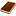國圖紙本論文推文當script無法執行時可按︰推文 網路書籤當script無法執行時可按︰網路書籤 推薦當script無法執行時可按︰推薦 評分當script無法執行時可按︰評分 引用網址當script無法執行時可按︰引用網址 轉寄當script無法執行時可按︰轉寄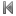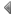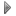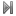top
 1 星狀網路通過特定邊的漢米爾頓迴圈與漢米爾頓路徑邊容錯之研究 2 建構「4正則、雙容錯之漢米爾頓圖形」的兩種方法 3 終端路徑覆蓋問題之研究 4 網格圖上漢米爾頓路徑之決定 5 映射對稱圖至超立方體衍生圖之錯誤避免法 6 圓弧圖與保距圖上的路徑覆誘峔銢袺鰤暋D 7 一般化煎餅網路之環狀容錯研究 8 有錯排置圖中漢彌頓路徑之嵌入 9 在特殊圖上一些問題的平行演算法設計及分析 10 路徑分割及相關問題 11 幾個特殊圖形上加權完美支配問題及其變形的多項式演算法 12 在區段圖與弦弧圖上對加權k-支配問題和其變形之有效率演算法

 1 吳姝蒨。2002。商業智慧的應用面向與成功導入關鍵要素。電子化企業經理人報告 (ARC Business Intelligence)，第5期，頁12-22。 2 吳明烈。2001。知識管理的概念、策略及其對學校組織的啟示。成人教育，第63期，頁12-22。 3 林銓鋃。2000。建置入口網站創造競爭新優勢。商業現代化，頁45。 4 林銓鋃。2000。建置入口網站創造競爭新優勢。商業現代化，頁45。 5 吳明烈。2001。知識管理的概念、策略及其對學校組織的啟示。成人教育，第63期，頁12-22。 6 吳姝蒨。2002。商業智慧的應用面向與成功導入關鍵要素。電子化企業經理人報告 (ARC Business Intelligence)，第5期，頁12-22。 7 譚大純。2000。知識管理文獻之回顧與前膽：以知識作業及知識策略主要分類點。管理評論，第20卷，第4期，頁 93-136。 8 譚大純。2000。知識管理文獻之回顧與前膽：以知識作業及知識策略主要分類點。管理評論，第20卷，第4期，頁 93-136。

 1 圓弧圖與保距圖上的路徑覆誘峔銢袺鰤暋D 2 在圓弧圖形與多重堆疊上的演算法 3 標記支配及其相關問題 4 資訊隱藏技術之研究 5 無線個人區域網路之有效能源感測暨服務品質保證繞徑協定 6 最短路徑演算法實作 7 中文文件自動分類方法的設計與評估 8 不同資料類型的有效壓縮法之研究 9 基於非一致性快取記憶架構與單周期環狀網路之多核處理器設計及其多層堆疊FPGA之雛型實現 10 適用於可變延遲處理器的多週期資料路徑合成方法 11 具高效能及簡略時間之交易性互聯模型與應用於PACDuo虛擬平台之案例 12 多核心平台軟體除錯架構與模擬加速 13 於多回合贊助商搜尋拍賣中設計適應式最小加價策略 14 利用快取機制來提升被動關聯式分類的效率 15 特定領域知識應用在中文文字分類的研究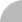簡易查詢 | 進階查詢 | 熱門排行 | 我的研究室